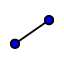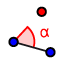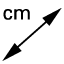# Draw transformations

## Drawing A Rotation

We are going to "draw" the 140o counter clockwise rotation of Triangle ABC around the origin. Rotate point A 1. Using the segment tooldraw a segment from Point A to the origin.
• Using settings make the segment a grey -------- segment.
2. Using the Angle with a given size iconmake a 140 degree angle. Select Point A then the origin, type in 140 and make sure counter clockwise is checked off. 3. Using the segment tool ﻿draw a segment from the origin Point A' (should of appeared after angle was formed).
• Using settings make the segment a grey -------- segment.
4. Using the Distance tool, find the length of OA and OA'
• When using the distance tool after clicking the icon select the endpoints of the segment you woud like to measure.
• What do you notice about the length of these segments?
5. A' is the rotated image of Point A.
• Go to the left side of the screen and start to check off the circles (so it is NOT colored in) of the commands so you ONLY see Triangle ABC and Point A'.
6. Repeat Steps 1-5 to Rotate Point B and Point C. 7. Check you work by using the "Rotate Around a Point"function.
• Select (click) Triangle ABC, then Origin, then tpye in 140 and make sure counter clockwise is checked
• Check to see if the rotated image MAPS onto your rotated A', B', and C' points.